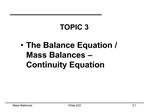# The General Balance Equation - PowerPoint PPT PresentationDownload PresentationThe General Balance Equation

The General Balance Equation
Download Presentation## The General Balance Equation

- - - - - - - - - - - - - - - - - - - - - - - - - - - E N D - - - - - - - - - - - - - - - - - - - - - - - - - - -
##### Presentation Transcript

1. Mass Balances Chee 223 3.1

2. Mass Balances Chee 223 3.2

3. Mass Balances Chee 223 3.3

4. Mass Balances Chee 223 3.4

5. Mass Balances Chee 223 3.5

6. Mass Balances Chee 223 3.6 The Mass Balance The law of conservation of mass states that mass may be neither created nor destroyed. With respect to a control volume (CV):

7. Mass Balances Chee 223 3.7 Conservation of Mass- Continuity Equation

8. Mass Balances Chee 223 3.8 Steady and Unsteady Flows The fluid flow in general is a function of location and time f(x, y, z, t). If the flow at every point in the fluid is independent of time, the flow is at steady state conditions. For a steady-state flow: dX/dt = 0, where X is a physical quantity (for example mass, temperature or velocity)

9. Mass Balances Chee 223 3.9 Mass and Volumetric Flow Rates Recall from definition of density:

10. Mass Balances Chee 223 3.10 Conservation of Mass- Continuity Equation In the general case for flows inside pipes the velocity is not the same at every point along the cross section of a pipe:

11. Mass Balances Chee 223 3.11 Conservation of Mass- Continuity Equation

12. Mass Balances Chee 223 3.12 Conservation of Mass- Continuity Equation

13. Mass Balances Chee 223 3.13 Example: Flow through an expansion A pipe carrying a liquid at 30 L/s, changes from a 15 cm ID (Internal Diameter) to a 20 cm ID. Find the velocities in each pipe

14. Mass Balances Chee 223 3.14 Conservation of Mass- Continuity Equation

15. Mass Balances Chee 223 3.15 Conservation of Mass- Continuity Equation

16. Mass Balances Chee 223 3.16 Example: Unsteady-State Mass Balance The tank shown in the figure has a volume V=1 m3 and contains air that is maintained at a constant temperature by being in thermal equilibrium with it surroundings. If the initial absolute pressure is Po=1 bar, how long will it take for the pressure to fall to a final pressure of 0.0001 bar if the air is evacuated at a constant rate of Q=0.001 m3/s?

17. Mass Balances Chee 223 3.17 Summary The first of the fundamental laws of fluid flow, the conservation of mass, has been considered. It is also called the continuity equation. The continuity equation has been derived using the �Control Volume�, or �integral method of analysis�, by applying the general balance equation to a finite control volume. Significant simplification of the continuity equation can be made by using the steady state and incompressibility assumptions.